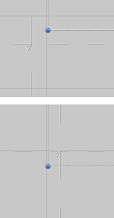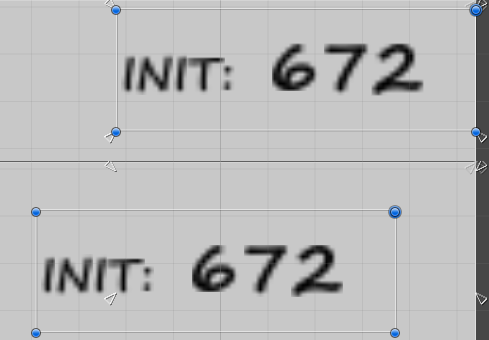# How To Make Anchor Snap To Own Rect Transform In Editor

How can I make the anchor points easily and accurately snap to the bounds of an objects rect transform? Where “object” is the object that the anchor points are being adjusted on? It seems that this is a regular thing people try to do, only, it never lines up quire right.Also, how can I move a rect transform and the anchor points for the same object at the same time? I can resize the both at the same time, but how can I reposition the rect transform and have the anchors follow?Thanks

It finally occurred to me that I could make some “edit mode” scripts that did both these things. I’ve included them below, feel free to use them. The features of the script are:

• Snap anchors to corners of Rect
• Snap anchors to corners when re-sizing or moving (both via mouse dragging)
• X, Y, Width, Height variables shown for editing (X, Y control the pivot location, irrelevant to the current anchors)
• Custom anchor X, Y (as a single point) relative to parent, to substitute for anchor positions being in corners.

anchorTool.cs

``````using UnityEngine;
using System.Collections;
[ExecuteInEditMode]
public class anchorTool : MonoBehaviour {
public bool manualRefresh = true;
public Rect anchorRect;
public Vector2 anchorVector;
private Rect anchorRectOld;
private Vector2 anchorVectorOld;
private RectTransform ownRectTransform;
private RectTransform parentRectTransform;
private Vector2 pivotOld;
private Vector2 offsetMinOld;
private Vector2 offsetMaxOld;

void Update () {
#if UNITY_EDITOR
ownRectTransform = gameObject.GetComponent<RectTransform>();
parentRectTransform = transform.parent.gameObject.GetComponent<RectTransform>();
if (ownRectTransform.offsetMin != offsetMinOld || ownRectTransform.offsetMax != offsetMaxOld) {
CalculateCurrentWH();
CalculateCurrentXY();
}
if (ownRectTransform.pivot != pivotOld || anchorVector != anchorVectorOld) {
CalculateCurrentXY();
pivotOld = ownRectTransform.pivot;
anchorVectorOld = anchorVector;
}
if (anchorRect != anchorRectOld) {
AnchorsToCorners();
anchorRectOld = anchorRect;
}
if (manualRefresh) {
manualRefresh = false;
CalculateCurrentWH();
CalculateCurrentXY();
AnchorsToCorners();
}
#endif
}

public void StopDrag() {
CalculateCurrentWH();
CalculateCurrentXY();
AnchorsToCorners();
}

private void CalculateCurrentXY() {
float pivotX = anchorRect.width * ownRectTransform.pivot.x;
float pivotY = anchorRect.height * (1 - ownRectTransform.pivot.y);
Vector2 newXY = new Vector2(ownRectTransform.anchorMin.x * parentRectTransform.rect.width + ownRectTransform.offsetMin.x + pivotX - parentRectTransform.rect.width * anchorVector.x,
- (1 - ownRectTransform.anchorMax.y) * parentRectTransform.rect.height + ownRectTransform.offsetMax.y - pivotY + parentRectTransform.rect.height * (1 - anchorVector.y));
anchorRect.x = newXY.x;
anchorRect.y = newXY.y;
anchorRectOld = anchorRect;
}

private void CalculateCurrentWH() {
anchorRect.width = ownRectTransform.rect.width;
anchorRect.height = ownRectTransform.rect.height;
anchorRectOld = anchorRect;
}

private void AnchorsToCorners() {
float pivotX = anchorRect.width * ownRectTransform.pivot.x;
float pivotY = anchorRect.height * (1 - ownRectTransform.pivot.y) ;
ownRectTransform.anchorMin = new Vector2(0f, 1f);
ownRectTransform.anchorMax = new Vector2(0f, 1f);
ownRectTransform.offsetMin = new Vector2(anchorRect.x / ownRectTransform.localScale.x, anchorRect.y / ownRectTransform.localScale.y - anchorRect.height);
ownRectTransform.offsetMax = new Vector2(anchorRect.x / ownRectTransform.localScale.x + anchorRect.width, anchorRect.y / ownRectTransform.localScale.y);
ownRectTransform.anchorMin = new Vector2(ownRectTransform.anchorMin.x + anchorVector.x + (ownRectTransform.offsetMin.x - pivotX) / parentRectTransform.rect.width * ownRectTransform.localScale.x,
ownRectTransform.anchorMin.y - (1 - anchorVector.y) + (ownRectTransform.offsetMin.y + pivotY) / parentRectTransform.rect.height * ownRectTransform.localScale.y);
ownRectTransform.anchorMax = new Vector2(ownRectTransform.anchorMax.x + anchorVector.x + (ownRectTransform.offsetMax.x - pivotX) / parentRectTransform.rect.width * ownRectTransform.localScale.x,
ownRectTransform.anchorMax.y - (1 - anchorVector.y) + (ownRectTransform.offsetMax.y + pivotY) / parentRectTransform.rect.height * ownRectTransform.localScale.y);
ownRectTransform.offsetMin = new Vector2((0 - ownRectTransform.pivot.x) * anchorRect.width * (1 - ownRectTransform.localScale.x), (0 - ownRectTransform.pivot.y) * anchorRect.height * (1 - ownRectTransform.localScale.y));
ownRectTransform.offsetMax = new Vector2((1 - ownRectTransform.pivot.x) * anchorRect.width * (1 - ownRectTransform.localScale.x), (1 - ownRectTransform.pivot.y) * anchorRect.height * (1 - ownRectTransform.localScale.y));

offsetMinOld = ownRectTransform.offsetMin;
offsetMaxOld = ownRectTransform.offsetMax;
}
}

//X and Y set the position of the Pivot relative to the parent Rect
//Anchor X and Y set where on the parent Rect the Pivot is relative to
//Where (0, 0) is the bottom left corner of parent Rect and (1, 1) the top right
``````

anchorToolEditor.cs

``````using UnityEngine;
using System.Collections;
using UnityEditor;

[CustomEditor(typeof(anchorTool))]
class anchorToolEditor : Editor {
void OnSceneGUI() {
if (Event.current.type == EventType.MouseUp && Event.current.button == 0) {
anchorTool myTarget = (anchorTool)target;
myTarget.StopDrag();
}
}
}

//This script must be placed in a folder called "Editor" in the root of the "Assets"
//Otherwise the script will not work as intended
``````

@ Phedg1 , I took your code and made a more convenient version.
One editor script only, will do the job for all the rectTransforms in the scene.

``````using UnityEngine;
using System.Collections;
using UnityEditor;
using UnityEngine;

public class AnchorToolsEditor : EditorWindow
{
static AnchorToolsEditor()
{
SceneView.onSceneGUIDelegate += OnScene;
}

private static void OnScene(SceneView sceneview)
{
if (Event.current.type == EventType.MouseUp && Event.current.button == 0)
{
UpdateAnchors();
}
}

public void OnDestroy()
{
SceneView.onSceneGUIDelegate -= OnScene;
}

static public Rect anchorRect;
static public Vector2 anchorVector;
static private Rect anchorRectOld;
static private Vector2 anchorVectorOld;
static private RectTransform currentRectTransform;
static private RectTransform parentRectTransform;
static private Vector2 pivotOld;
static private Vector2 offsetMinOld;
static private Vector2 offsetMaxOld;

static public void UpdateAnchors()
{
TryToGetRectTransform();
if (currentRectTransform != null && parentRectTransform != null && ShouldStick())
{
//Debug.Log("[Anchors Tools] Updating");
Stick();
}
}

static private bool ShouldStick()
{
return (
currentRectTransform.offsetMin != offsetMinOld ||
currentRectTransform.offsetMax != offsetMaxOld ||
currentRectTransform.pivot != pivotOld ||
anchorVector != anchorVectorOld ||
anchorRect != anchorRectOld
);
}

static private void Stick()
{
CalculateCurrentWH();
CalculateCurrentXY();

CalculateCurrentXY();
pivotOld = currentRectTransform.pivot;
anchorVectorOld = anchorVector;

AnchorsToCorners();
anchorRectOld = anchorRect;

UnityEditor.EditorUtility.SetDirty(currentRectTransform.gameObject);
}

static private void TryToGetRectTransform()
{
currentRectTransform = UnityEditor.Selection.activeGameObject.GetComponent<RectTransform>();
parentRectTransform = currentRectTransform.parent.gameObject.GetComponent<RectTransform>();

}

static private void CalculateCurrentXY()
{
float pivotX = anchorRect.width * currentRectTransform.pivot.x;
float pivotY = anchorRect.height * (1 - currentRectTransform.pivot.y);
Vector2 newXY = new Vector2(currentRectTransform.anchorMin.x * parentRectTransform.rect.width + currentRectTransform.offsetMin.x + pivotX - parentRectTransform.rect.width * anchorVector.x,
-(1 - currentRectTransform.anchorMax.y) * parentRectTransform.rect.height + currentRectTransform.offsetMax.y - pivotY + parentRectTransform.rect.height * (1 - anchorVector.y));
anchorRect.x = newXY.x;
anchorRect.y = newXY.y;
anchorRectOld = anchorRect;
}

static private void CalculateCurrentWH()
{
anchorRect.width = currentRectTransform.rect.width;
anchorRect.height = currentRectTransform.rect.height;
anchorRectOld = anchorRect;
}

static private void AnchorsToCorners()
{
float pivotX = anchorRect.width * currentRectTransform.pivot.x;
float pivotY = anchorRect.height * (1 - currentRectTransform.pivot.y);
currentRectTransform.anchorMin = new Vector2(0f, 1f);
currentRectTransform.anchorMax = new Vector2(0f, 1f);
currentRectTransform.offsetMin = new Vector2(anchorRect.x / currentRectTransform.localScale.x, anchorRect.y / currentRectTransform.localScale.y - anchorRect.height);
currentRectTransform.offsetMax = new Vector2(anchorRect.x / currentRectTransform.localScale.x + anchorRect.width, anchorRect.y / currentRectTransform.localScale.y);
currentRectTransform.anchorMin = new Vector2(currentRectTransform.anchorMin.x + anchorVector.x + (currentRectTransform.offsetMin.x - pivotX) / parentRectTransform.rect.width * currentRectTransform.localScale.x,
currentRectTransform.anchorMin.y - (1 - anchorVector.y) + (currentRectTransform.offsetMin.y + pivotY) / parentRectTransform.rect.height * currentRectTransform.localScale.y);
currentRectTransform.anchorMax = new Vector2(currentRectTransform.anchorMax.x + anchorVector.x + (currentRectTransform.offsetMax.x - pivotX) / parentRectTransform.rect.width * currentRectTransform.localScale.x,
currentRectTransform.anchorMax.y - (1 - anchorVector.y) + (currentRectTransform.offsetMax.y + pivotY) / parentRectTransform.rect.height * currentRectTransform.localScale.y);
currentRectTransform.offsetMin = new Vector2((0 - currentRectTransform.pivot.x) * anchorRect.width * (1 - currentRectTransform.localScale.x), (0 - currentRectTransform.pivot.y) * anchorRect.height * (1 - currentRectTransform.localScale.y));
currentRectTransform.offsetMax = new Vector2((1 - currentRectTransform.pivot.x) * anchorRect.width * (1 - currentRectTransform.localScale.x), (1 - currentRectTransform.pivot.y) * anchorRect.height * (1 - currentRectTransform.localScale.y));

offsetMinOld = currentRectTransform.offsetMin;
offsetMaxOld = currentRectTransform.offsetMax;
}
}
////This script must be placed in a folder called "Editor" in the root of the "Assets"
////Otherwise the script will not work as intended
``````

Based on the solution here, I created some MenuItem that will perform “move anchors to corners” via keyboard shortcut: Unity3D Editor script that adds MenuItem to move the anchors of a RectTransform to its corners. · GitHub

You might also be interested in the other way around, i.e., “move corners to anchors”: Unity3D Editor script that adds some MenuItem to move the corners of a RectTransform to its anchors. · GitHub

this is what you are looking for.
thank me later